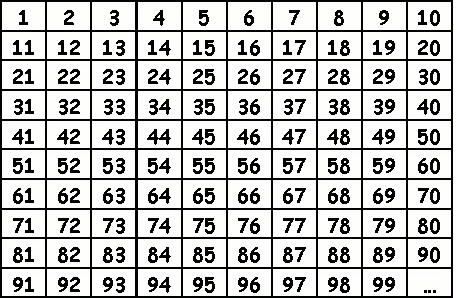HELP MATHS

PRIME NUMBERS
Return

WHAT IS A PRIME NUMBER?
A prime number is a positive integer (natural number) that has exactly two factors, 1 and itself.

1 is not a prime number, it has ONLY one factor.

HOW CAN YOU FIND PRIME NUMBERS?
Step by stepThe rule for working out prime numbers is really simple, but there is not a formula for telling you if a big
number is a prime number. If a number is really big it can take years to work out if it is a prime number.